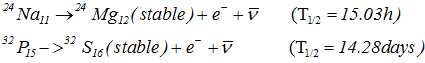# Beta decay|gamma decay

### 6) β Decay

• There are two types of β decay , β- and β+
• In β decay an nucleus decay spontaneously emitting an electron or positron
• Under β- decay one of the neutrons in the parent nucleus gets transformed into a proton and in the process an electron and an antineutrino are emitted
n-> p+e--
• The daughter nucleus thus formed in β- decay would be an element one place to the right of the parent in the periodic table of elements
• Examples of β- decay• β- is common over entire range of nuclides and amongst the naturally occurring heavy radioactive nuclides and in fission products
• In β+ decay one the protons of the parent nucleus gets transformed into a neutron emitting a positron and neutrino
p->n+e+
• In β+ decay the daughter nucleus would be one place to the left of parent nuclei in the periodic table
• Examples of β+ decay• In both β+ and β- symbol ν- and ν represents antineutrino and neutrino
• Both antineutrino (ν-) and neutrino(ν) are charge less and nearly less particles and interact very weakly with matter which make their detection very difficult

• In these β decay( β+ and β-) mass number A of nucleus remain same after the decay

### 7) γ Decay

• After alpha or beta decay processes it is common to find the daughter nucleus to be in an excited state
• Just like atoms ,nucleus also have energy levels
• So an nucleus in excited state can make transitions from higher energy levels to lower one by the emission of electro magnetic radiation
• The energy difference in allowed energy levels of a nucleus are of the order of Mev and the photons emitted by nuclei have energies of the order of Mev and are called γ rays
• As an example,β decay of 60Co27 nucleus gets transformed into 60Ni28 nucleus in excited state which then de -excites to its ground state by successive emission of 1.17 Mev and 1.33 Mev gamma rays as shown in energy level diagram,